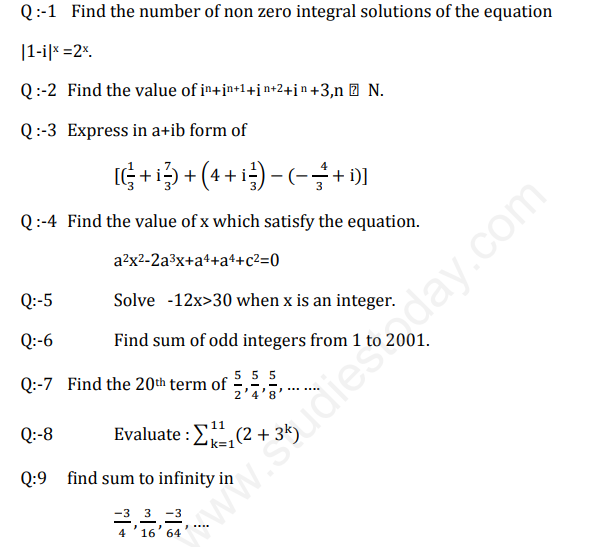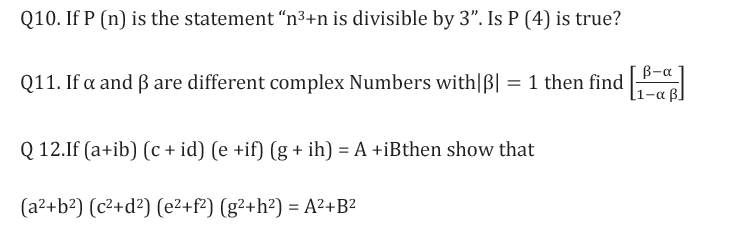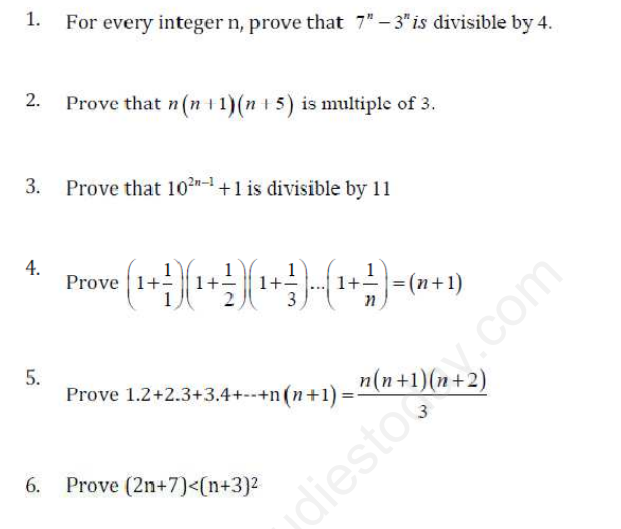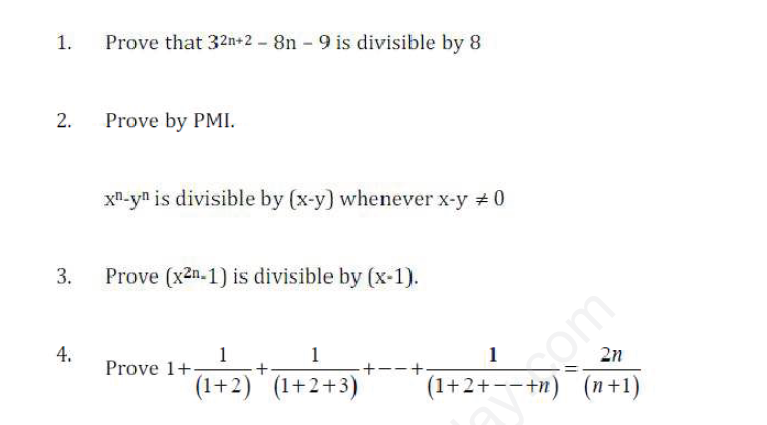# Mathematics Class 11 Assignments

• ### CBSE Class 11 Mathematics Three Dimensional Geometry Assignment Set A

Q1. (i) If (x-y, 3) = (5, x+y) then find the value of x and y. Ans. 4,-1, (ii) If (x+3, 5) = (6, 2x+y) then find the value of x and y. Ans. 3,-1, (iii) If (x-1, y+3) = (2, -5) then find the value of x and y. Ans. 3,-8,   Q2. If n (A) =p, n (B) =q, n(C) = r and n (D) = s then find (i) n (AxBxCxD...

• ### CBSE Class 11 Mathematics Revision Assignment Set A

Please click the below link to access CBSE Class 11 Mathematics Revision Assignment Set A

• ### CBSE Class 11 Mathematics Revision Assignment Set B

Please click the below link to access CBSE Class 11 Mathematics Revision Assignment Set B

• ### CBSE Class 11 Mathematics Revision Assignment Set C

Please click the below link to access CBSE Class 11 Mathematics Revision Assignment Set C

• ### CBSE Class 11 Mathematics Revision Assignment Set D

4. (i) If P(2n – 1, n) : P (2n + 1, n – 1) = 22 : 7, find n (ii) If P(2n , 3) : P (n,3) = 11 : 1, find n 5. How many numbers are there between 100 and 1000 which have exactly one of their digits as 7? 6. How many words beginning and ending with a consonant can be formed by using the letters of...

# CBSE Class 11 Mathematics Three Dimensional Geometry Assignment Set A

Q1. (i) If (x-y, 3) = (5, x+y) then find the value of x and y.

Ans. 4,-1,

(ii) If (x+3, 5) = (6, 2x+y) then find the value of x and y.

Ans. 3,-1,

(iii) If (x-1, y+3) = (2, -5) then find the value of x and y.

Ans. 3,-8,

Q2. If n (A) =p, n (B) =q, n(C) = r and n (D) = s then find

(i) n (AxBxCxD)

(ii) Number of possible subset of (AxBxCxD)

Click for more Introduction of 3D Study Material
 CBSE Class 11 Mathematics Three Dimensional Geometry Assignment Set B CBSE Class 11 Mathematics Three Dimensional Geometry Assignment Set C CBSE Class 11 Mathematics Three Dimensional Geometry Assignment Set A

# CBSE Class 11 Mathematics Revision Assignment Set AClick for more Mathematics Study Material
 CBSE Class 11 Mathematics Revision Assignment Set C CBSE Class 11 Mathematics Revision Assignment Set B CBSE Class 11 Mathematics Revision Assignment Set A CBSE Class 11 Mathematics Revision Assignment Set G CBSE Class 11 Mathematics Revision Assignment Set F CBSE Class 11 Mathematics Revision Assignment Set E CBSE Class 11 Mathematics Revision Assignment Set D

# CBSE Class 11 Mathematics Revision Assignment Set BClick for more Mathematics Study Material
 CBSE Class 11 Mathematics Revision Assignment Set C CBSE Class 11 Mathematics Revision Assignment Set B CBSE Class 11 Mathematics Revision Assignment Set A CBSE Class 11 Mathematics Revision Assignment Set G CBSE Class 11 Mathematics Revision Assignment Set F CBSE Class 11 Mathematics Revision Assignment Set E CBSE Class 11 Mathematics Revision Assignment Set D

# CBSE Class 11 Mathematics Revision Assignment Set CPlease click the below link to access CBSE Class 11 Mathematics Revision Assignment Set C

Click for more Mathematics Study Material
 CBSE Class 11 Mathematics Revision Assignment Set C CBSE Class 11 Mathematics Revision Assignment Set B CBSE Class 11 Mathematics Revision Assignment Set A CBSE Class 11 Mathematics Revision Assignment Set G CBSE Class 11 Mathematics Revision Assignment Set F CBSE Class 11 Mathematics Revision Assignment Set E CBSE Class 11 Mathematics Revision Assignment Set D

# CBSE Class 11 Mathematics Revision Assignment Set D4. (i) If P(2n – 1, n) : P (2n + 1, n – 1) = 22 : 7, find n

(ii) If P(2n , 3) : P (n,3) = 11 : 1, find n

5. How many numbers are there between 100 and 1000 which have exactly one of their digits as 7?

6. How many words beginning and ending with a consonant can be formed by using the letters of the word EQUATION?

Click for more Mathematics Study Material
 CBSE Class 11 Mathematics Revision Assignment Set C CBSE Class 11 Mathematics Revision Assignment Set B CBSE Class 11 Mathematics Revision Assignment Set A CBSE Class 11 Mathematics Revision Assignment Set G CBSE Class 11 Mathematics Revision Assignment Set F CBSE Class 11 Mathematics Revision Assignment Set E CBSE Class 11 Mathematics Revision Assignment Set D

# CBSE Class 11 Mathematics Revision Assignment Set E

CBSE Class 11 Mathematics Revision Assignment Set E . Based on CBSE and NCERT guidelines. The students should read these basic concepts to gain perfection which will help him to get more marks in CBSE examination.

Section - A

Click for more Mathematics Study Material
 CBSE Class 11 Mathematics Revision Assignment Set C CBSE Class 11 Mathematics Revision Assignment Set B CBSE Class 11 Mathematics Revision Assignment Set A CBSE Class 11 Mathematics Revision Assignment Set G CBSE Class 11 Mathematics Revision Assignment Set F CBSE Class 11 Mathematics Revision Assignment Set E CBSE Class 11 Mathematics Revision Assignment Set D

# CBSE Class 11 Mathematics Revision Assignment Set F

Click for more Mathematics Study Material
 CBSE Class 11 Mathematics Revision Assignment Set C CBSE Class 11 Mathematics Revision Assignment Set B CBSE Class 11 Mathematics Revision Assignment Set A CBSE Class 11 Mathematics Revision Assignment Set G CBSE Class 11 Mathematics Revision Assignment Set F CBSE Class 11 Mathematics Revision Assignment Set E CBSE Class 11 Mathematics Revision Assignment Set D

# CBSE Class 11 Mathematics Revision Assignment Set G

CBSE Class 11 Mathematics Revision Assignment Set G . Based on CBSE and NCERT guidelines. The students should read these basic concepts to gain perfection which will help him to get more marks in CBSE examination.

Chapter 1

Click for more Mathematics Study Material
 CBSE Class 11 Mathematics Revision Assignment Set C CBSE Class 11 Mathematics Revision Assignment Set B CBSE Class 11 Mathematics Revision Assignment Set A CBSE Class 11 Mathematics Revision Assignment Set G CBSE Class 11 Mathematics Revision Assignment Set F CBSE Class 11 Mathematics Revision Assignment Set E CBSE Class 11 Mathematics Revision Assignment Set D

# CBSE Class 11 Mathematics Three Dimensional Geometry Assignment Set C

Click for more Introduction of 3D Study Material
 CBSE Class 11 Mathematics Three Dimensional Geometry Assignment Set B CBSE Class 11 Mathematics Three Dimensional Geometry Assignment Set C CBSE Class 11 Mathematics Three Dimensional Geometry Assignment Set A

## Latest NCERT & CBSE News

Read the latest news and announcements from NCERT and CBSE below. Important updates relating to your studies which will help you to keep yourself updated with latest happenings in school level education. Keep yourself updated with all latest news and also read articles from teachers which will help you to improve your studies, increase motivation level and promote faster learning

### MCQ Question based CBSE examination

For 2021-22 CBSE has launched MCQ question-based examination for Term 1 & Term 2 board examinations. The entire syllabus has been divided into two parts each including 50% of the entire syllabus. To score well, students must practice as per the new CBSE term-wise...

### CBSE Board Examination Scheme of Assessment 2021 2022

A. Academic session to be divided into 2 Terms with approximately 50% syllabus in each term: The syllabus for the Academic session 2021-22 will be divided into 2 terms by following a systematic approach by looking into the interconnectivity of concepts and topics by...

### CBSE Class 10 Revised Syllabus

Last year CBSE had to reduce the syllabus because of the pandemic situation but it was not very effective because there were no examinations. This year to avoid any confusion and conflict, CBSE has decided to reduce the syllabus into term 1 and term 2. 50 percent of...

### How To Solve Unseen Passages In English

Unseen passages may contain one or many paragraphs. This is one of the important yet easy parts for a student to get marks. Students should thoroughly study and understand the passage to answer the related questions. The unseen passages are there just to test the...

### Score well in Class 12 English Boards Exam

12th Board exams are an important part of students' lives. The marks obtained in the board exam decide the college in which one can study. In class 12 the syllabus of each and every subject increases vastly and it is difficult to cover up every point. In English also...

### Moderation of Marks Class 11 and 12 Board Exams

The portal for moderation and finalization of results for Class-12 is being opened from 16.07.2021 to 22.07.2021. As Board has to declare the result latest by 31.07.2021, schools have been requested to follow the schedule strictly and complete the moderation within...

×